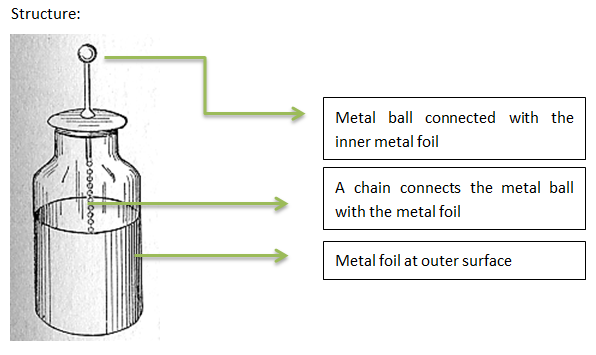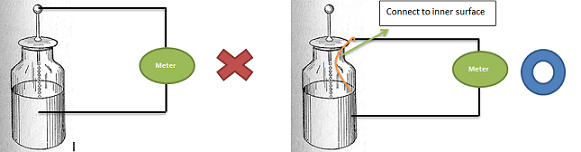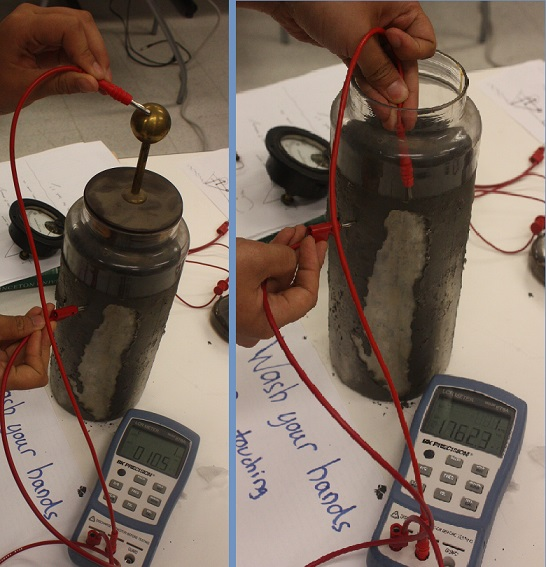# Leyden jar

Leyden jar: A Leyden jar, or Leiden jar, is a device that “stores” static electricity between two electrodes on the inside and outside of a glass jar [wikipedia]. It was widely used to conduct many early experiments in electricity. A typical design consists of a glass jar with conducting metal foil coating the inner and outer surfaces.It is necessary to measure the capacitance of a Leyden jar for estimation in experiments. However the capacitance cannot be measured directly. The reason is that the metal ball is connected through a chain, which provides conduction only while arcing. However it is hard to measure when arcing due to the high voltage. A possible approach is to connect the Leyden jar in a RC circuit and measure the time constant of discharge. However it is difficult in practice because the voltage of Leyden jar is very high(around 10kV) and it is hard to measure it using common equipment in lab. I tried some similar way such as using a large capacitor in parallel but unable to get reasonable result. Another possible way is to by-pass the chain:### Our measurement

We did some measurement with the Leyden jar used in later experiments following the method above:Result:
The measured result is 1800pF by direct connection. The Leyden jar’s capacitance can be calculated using the equation C=ԐA/d, where Ԑ is the absolute permittivity and Ԑ = Ԑr * Ԑ0, ε0 = 8.854187817.. × 10−12 F/m; A is the cross area of the capacitor and d is the gap between two conducting plates. Here the size of the jar is 21cm in height, 10.6cm in diameter and the thickness is around 2mm. The dielectric is glass and the relative permittivity for glass various from 3.7 to 10. Here we use 4.7 for calculation. The calculated result is therefore C=4.7 * 8.854187817 × 10−12 F/m * 10.6cm * pi * 21cm / 2mm = 1455pF. The calculated result agrees with the measured result.